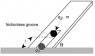# Ball Balanced by Forces

• Kaylee

## Homework Statement

A single charge q1 = 1.10E-7 C is fixed at the base of a plane that makes an angle theta with the horizontal direction. A small ball of mass m = 1.75 g and a charge q2 = 3.30E-8 C is placed into a smooth frictionless groove in the plane that extends directly to the fixed charge (image attached.) It is allowed to move up and down until it finds a stable position d = 11.0 cm from the fixed charge. What is the value of theta (t)?

Fe = kq1q2/d^2
Fg = mg

## The Attempt at a Solution

Fe = (8.99E9)(1.1E-7)(3.3E-8)/(0.11^2) = 0.002697 N [up to the right]
Fg = (.00175)(9.8) = 0.01715 N [down]

(Fe)(sin(t)) = mg
(0.002697)(sin(t)) = 0.01715
sin(t) = 6.358917316
Since sin(t) has to be between -1 and 1 it is impossible to find t.

Any help detecting my problem would be greatly appreciated.

#### Attachments

•Physics Image.png
4.6 KB · Views: 496
I think you forgot about the normal force.

Try to set up the vector decomposition differently.

(Fe)(sin(t)) = mg
That's wrong. Which direction are you resolving into get that?

I think you forgot about the normal force.

Try to set up the vector decomposition differently.
So my new equation would be
mg = Fe(sin(t)) + N.
I'm confused as to where to go from here.
What did you mean by "set up the vector decomposition differently."

That's wrong. Which direction are you resolving into get that?
I'm resolving along the y-axis (vertical). If you look at the photo, I think it is right.

You found the vertical component of Fe.

If you take the plane to be the x-axis, you can find the horizontal component of mg.

I'm resolving along the y-axis (vertical). If you look at the photo, I think it is right.
No, as you replied to showzen, it wasn't right because you had left out the normal force.
The simpler path, though, is to resolve parallel to the plane so that you don't need to involve the normal force.

No, as you replied to showzen, it wasn't right because you had left out the normal force.
The simpler path, though, is to resolve parallel to the plane so that you don't need to involve the normal force.
Sorry, when you said "That's wrong," I thought you meant that I got the direction, as in I used the wrong trig function.

Thanks for your help. Changing my coordinate system makes the whole problem much easier.

Sorry, when you said "That's wrong," I thought you meant that I got the direction, as in I used the wrong trig function.
I couldn't tell which - either you'd resolved vertically and omitted the normal force, or you'd resolved down the plane and put the sine factor on the wrong side. I've seen the second done more often than the first.

I couldn't tell which - either you'd resolved vertically and omitted the normal force, or you'd resolved down the plane and put the sine factor on the wrong side. I've seen the second done more often than the first.
Thanks again for all your help! This forum is very helpful to fully understand where I am going wrong.